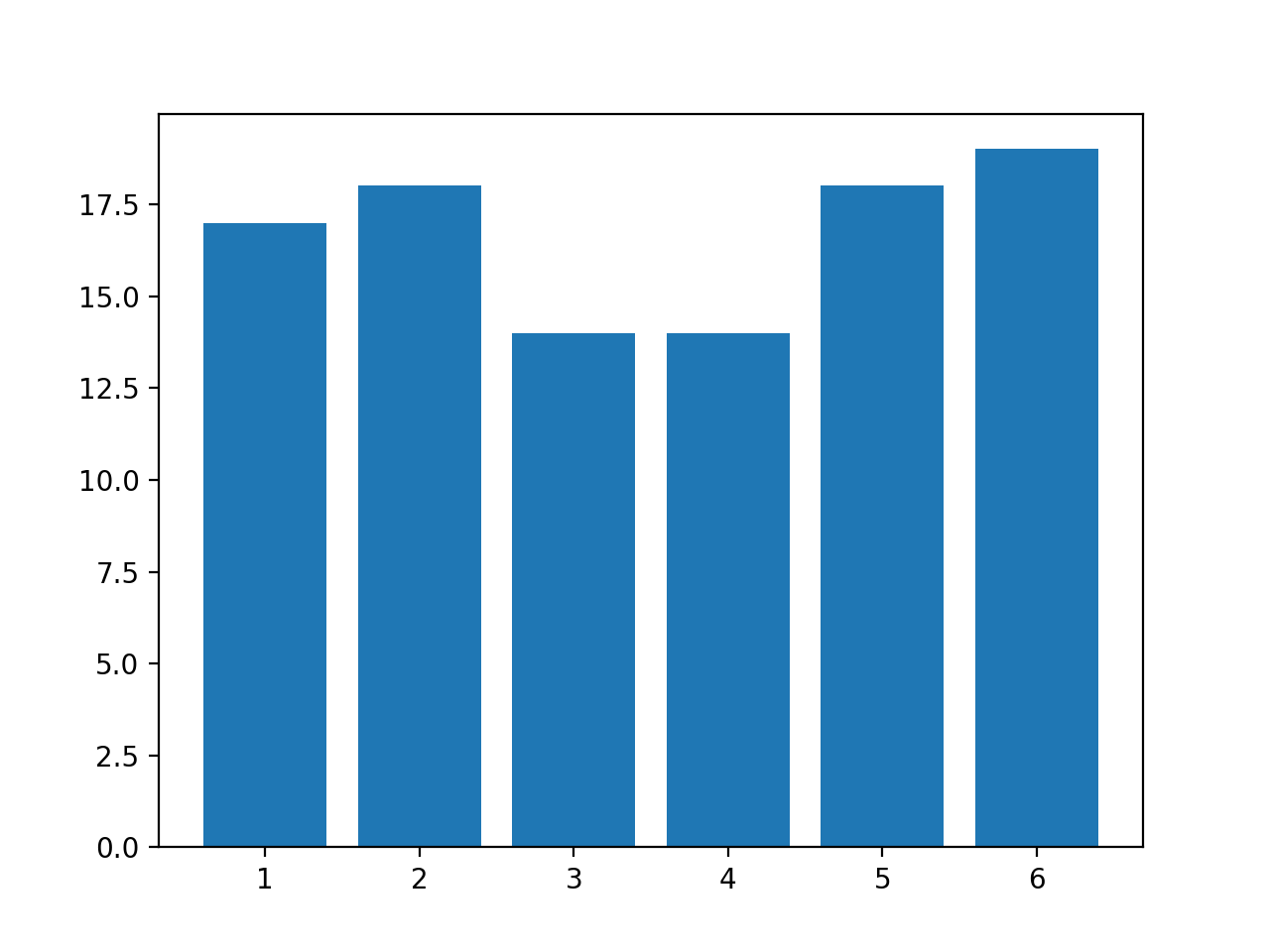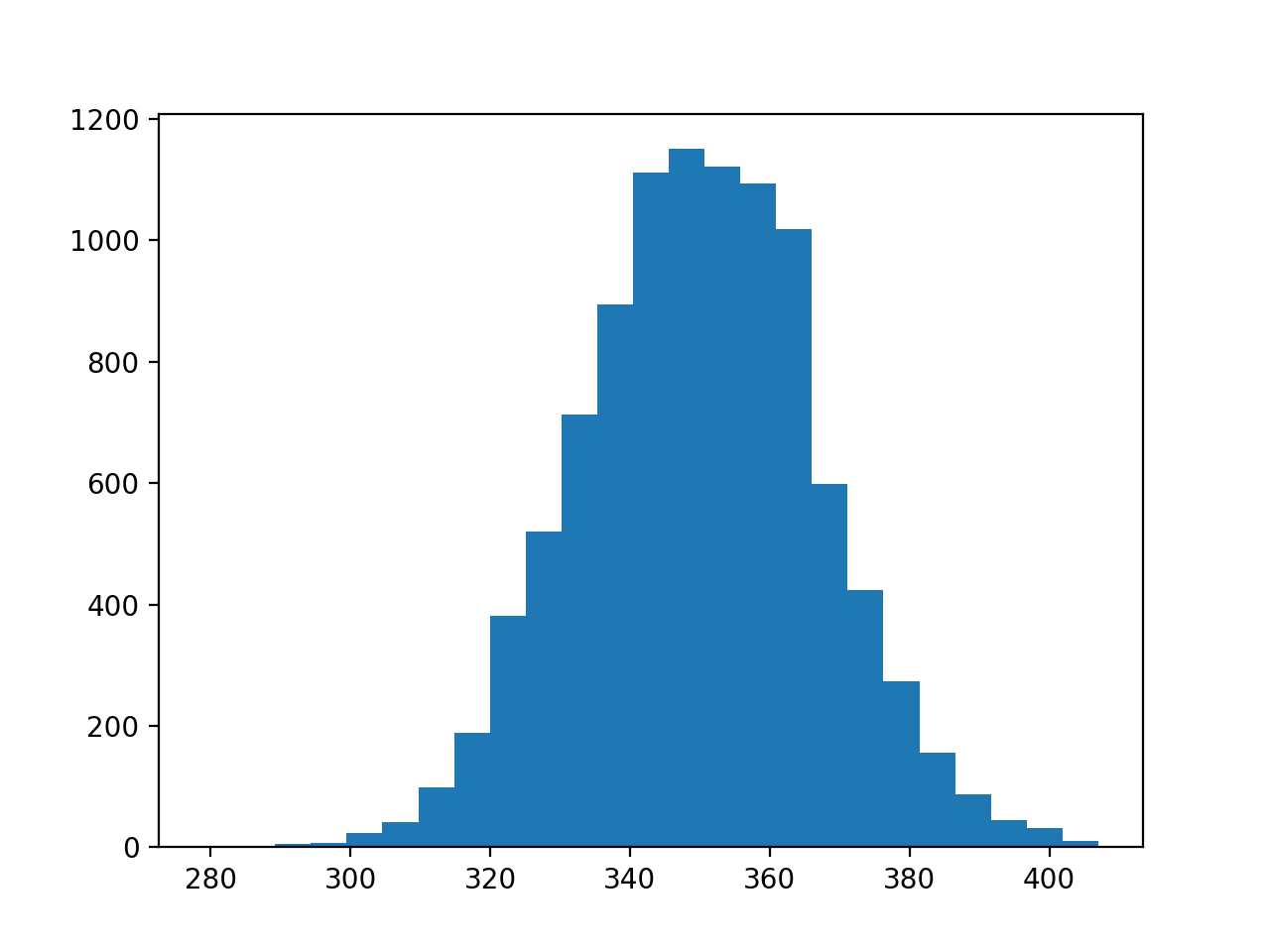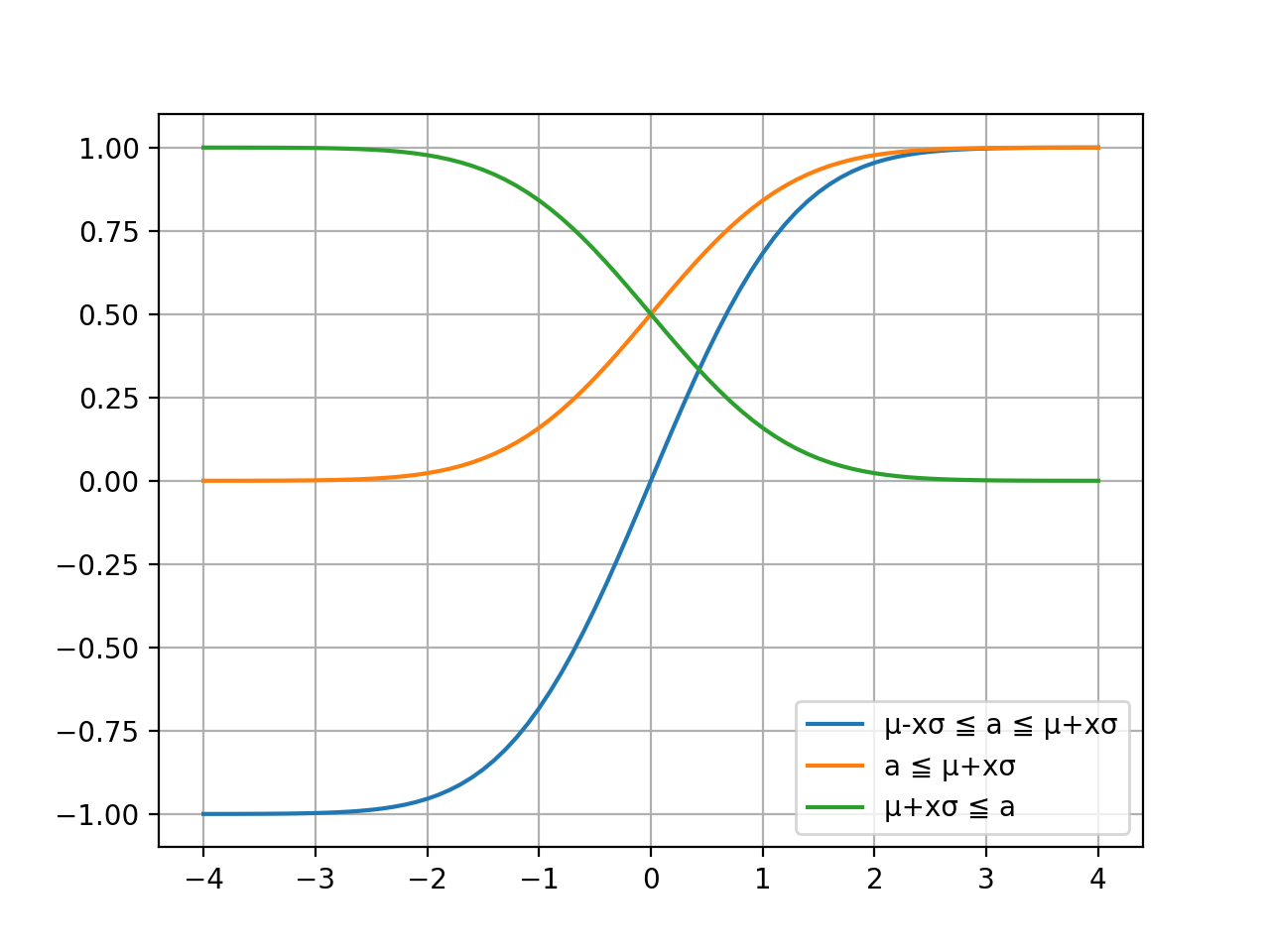# Question 1

What is the total number of rolls when you roll the dice 100 times?## Average / expected value

The average learned in elementary school is the top of the statistics. First, use the average to derive the answer to the first question and compare it with the simulation results.

### program

``````import numpy as np
import numpy.random as rd
import matplotlib.pyplot as plt

#Define the dice
#Know the average
dice = [1, 2, 3, 4, 5, 6]
print("average:", np.mean(dice))

#Define the number of attempts
#You can see the expected value of the total in combination with the average
trialsNum = 100
print("Total expected value:", np.mean(dice) * trialsNum)
input("Press Enter to continue. . .")

#Actually try
#Draw a histogram to check the distribution of rolls
resultList = [rd.choice(dice) for i in range(trialsNum)]
plt.hist(resultList, bins=6, rwidth=0.8, range=(0.5, 6.5))
plt.show()

print("total:", np.sum(resultList))
``````

### Execution result (example)

The result is not constant because it uses random numbers.

``````Sample mean: 3.5
Total expected value: 350.0
Press Enter to continue. . .
````````````total: 355
``````

### Commentary

The average number of rolls you can get when you roll the dice once is 3.5, so the total of 100 rolls is about 350, which is 100 times the average. You can manage the progress as follows by using the average / expected value.

1. Obtain the average (= progress pace) of the amount of tasks digested per resource unit (person day, etc.) from past results
2. Multiply the amount of resources remaining available by the due date by the pace of progress to find the expected amount of tasks that can be digested.
3. Compare the expected value with the remaining ** task amount that must be digested by the due date to determine the progress **

But is it okay to leave the comparison and judgment to human experience and intuition? You can use the burndown chart to make judgments by looking at trends over time, but you still rely on experience and intuition.

## Variance / standard deviation

About 350 ... You can answer the first question.

About 350 ... How much is "about 350"? The variance / standard deviation represents this. First, find the standard deviation from the sample, then find the standard deviation from the simulation results and compare them.

### program

``````import numpy as np
import numpy.random as rd
import matplotlib.pyplot as plt

#Define the dice
#Know the mean and variance
dice = [1, 2, 3, 4, 5, 6]
print("Sample mean:", np.mean(dice))
print("Sample variance:", np.var(dice))

#Define the number of attempts
#You can see the expected value of the total in combination with the sample mean
#Can predict total standard deviation in combination with sample variance
trialsNum = 100
print("Total expected value:", np.mean(dice) * trialsNum)
print("Total standard deviation (expected):", np.sqrt(np.var(dice) * trialsNum))
input("Press Enter to continue. . .")

#Actually try...Try
metaTrialsNum = 10000
resultList = [np.sum([rd.choice(dice) for i in range(trialsNum)])
for i in range(metaTrialsNum)]
myMean = np.mean(resultList)
myStd = np.std(resultList)
print("Average of total:", myMean)
print("Total standard deviation (actual):", myStd)

# 68–95–99.Check if the 7 rules apply
win = [len([n for n in resultList if myMean - r * myStd <= n and n <= myMean + r * myStd]) /
metaTrialsNum for r in range(1, 4)]
print(
f'μ±σ : {myMean - 1 * myStd :.1f} ~ {myMean + 1 * myStd:.1f}: {win:.2%}')
print(
f'μ±2σ: {myMean - 2 * myStd :.1f} ~ {myMean + 2 * myStd:.1f}: {win:.2%}')
print(
f'μ±3σ: {myMean - 3 * myStd :.1f} ~ {myMean + 3 * myStd:.1f}: {win:.2%}')

#Draw a histogram to see the total distribution
plt.hist(resultList, bins=25)
plt.show()
``````

### Execution result (example)

The result is not constant because it still uses random numbers.

``````Sample mean: 3.5
Sample variance: 2.9166666666666665
Total expected value: 350.0
Total standard deviation (expected): 17.078251276599328
Press Enter to continue. . .
````````````Average of total: 349.9814
Total standard deviation (actual): 17.034108548438923
μ±σ : 332.9 ~ 367.0: 69.69%
μ±2σ: 315.9 ~ 384.0: 95.77%
μ±3σ: 298.9 ~ 401.1: 99.76%
``````

### Commentary

The total distribution fits nicely into the 68–95–99.7 rule. It became a distribution. The standard deviation of about 17 shows how much it is "about 350". And the standard deviation can be obtained from the sample without simulation.

## Error function erf

From the 68–95–99.7 rule, we found that if x in μ ± xσ is 1,2,3, the probability that the trial result is within that range is found. So don't you know the probability when x is 1.5? Or do you know the probability that the total number of rolls will be 370 or more? That's where the error function erf comes in. Let's illustrate how this function works in the python program below.

### program

``````import math
import numpy as np
import matplotlib.pyplot as plt

x = np.arange(-4.0, 4.1, 0.1)

leg1 = "μ-xσ ≦ a ≦ μ+xσ"
y1 = [math.erf(i/math.sqrt(2)) for i in x]
p1 = plt.plot(x, y1)

leg2 = "a ≦ μ+xσ"
y2 = [0.5 + 0.5 * math.erf(i/math.sqrt(2)) for i in x]
p2 = plt.plot(x, y2)

leg3 = "μ+xσ ≦ a"
y3 = [0.5 - 0.5 * math.erf(i/math.sqrt(2)) for i in x]
p3 = plt.plot(x, y3)

plt.legend((p1, p2, p3),
(leg1, leg2, leg3), loc=0)
plt.grid(True)
plt.show()
``````

### Execution result### Commentary

• Blue line: Shows the probability that the trial result a is in the range μ ± xσ
• Orange line: Shows the probability that the trial result a is less than or equal to μ + xσ
• Green line: Shows the probability that the trial result a is greater than or equal to μ + xσ

The error function erf allows you to calculate the probability that the trial result is within μ ± xσ and the probability that it is less than or greater than μ + xσ for any x.

Or do you know the probability that the total number of rolls will be 370 or more?

It can be found using the error function erf First, find the value of x by applying the values to μ and σ in the following equation.

μ+xσ = 370

Total expected value: 350.0 Total standard deviation (estimated): 17.078251276599328

350+17x = 370 17x = 20 x = 1.18

You can then calculate the probability by applying a value to x in the expression used in your program.

`0.5 - 0.5 * math.erf(i/math.sqrt(2)`

0.5 - 0.5 * erf(1.18/√2) = 0.12 = 12%

When you roll the dice 100 times, there is a 12% chance that the total number of rolls will be 370 or higher. The mean, standard deviation, and error function allow us to answer the first question in many ways.

# Question 2

How far will the teams that have progressed at the pace shown in the table below progress when iteration 20 is completed?

Iteration Velocity Accumulation
1 7 7
2 3 10
3 3 13
4 6 19
5 6 25

## Average / expected value

μ = 200, isn't it?

## Variance / standard deviation

Since the variance up to iteration 5 is 3.5, the standard deviation up to iteration 20 can be expected to be σ = √ (3.5 * 20) ≒ 8.4. The range of μ ± 3σ is approximately 175 to 225.

## Error function erf

If you answer with an accuracy of about 80%, say 191 with μ-1σ (rounded down). If the progress goal up to iteration 20 is greater than that, you should negotiate to lower the goal to 191.

If you answer with 99% accuracy, say 175 with μ-3σ. If your goal is 225, there is no 1% chance that you will be in time. Even if the goal is 200, the probability of being in time is 50%, which is a fifty-fifty gambling. It's easy to get hurt when you judge that "If you keep going at the average pace, you'll be in time!"

Since both μ and σ fluctuate as the achievements of progress are accumulated, let's calculate the probability of progressing beyond the target with the error function erf at any time. Unlike dice, there is no perfect specimen.

The unit of resource amount is easy if it is the number of iterations, but if you want to make it more detailed, you can also calculate it by the number of days or man-days.